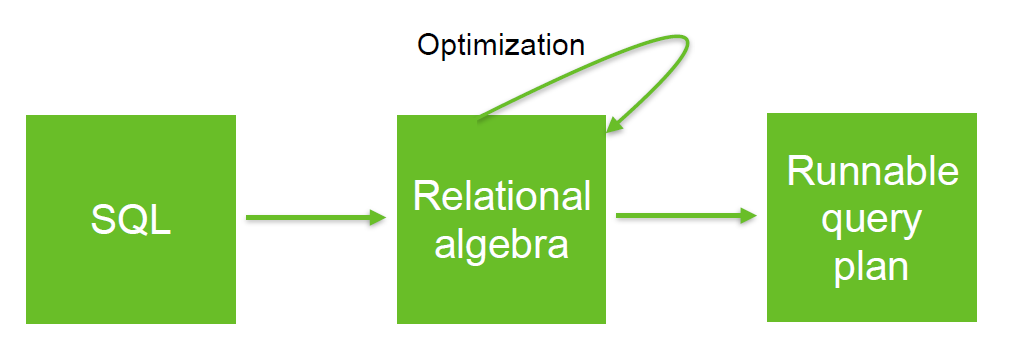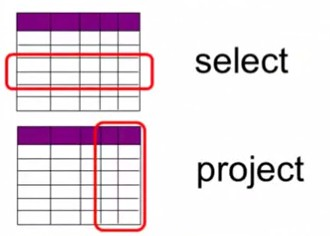# Relational Algebra - Select Operator (Selection) -

The select operator is a relational operator that select the rows of a relation.

## Visualisation

select vs project

## Notation

• Keep rows that satisfy the condition c (ie predicate)
• Delete rows that fail the condition c (ie predicate)

## Example

Select the shirts that satisfy the condition color=blue

• Relational Algebra Notation

$$\LARGE \sigma_{(color='Blue')} Shirts$$

• SQL Notation
SELECT * FROM Shirts WHERE color='Blue'


Discover MoreLogical Data Modeling - Duplicate

A duplicate happens when in a set two elements have an equivalence relationship that returns true. In the set {1,1,2,3}, 1 is a duplicate The set {1,2,3} has no duplicate relational operators...Relational Algebra - Expression and Operators

Relational algebra is based upon the fact that you can pass tabular data through a set of data operators (select, filter, join, sort, union, etc.) in a algebraic structure. It means that: the output...Relational Algebra - Project operator (Projection)

The project operator is a relational operator that keeps: only a set of columns (in other words eliminates the others). or delete attributes not in the projection list It chooses a subset of the...Relational Algebra - Union operator

The union operation will takes two relations that are union-compatible (ie both relations have the same number of fields with the same types and returns all Tuples appearing in either relation (in Rel...Relational Operator - Equi-joins

An Equi-join is a join where the condition (predicate) is an equality. theta join An equi-join links two relations (tables, ...) on common values in a column in relation 1 with a column in relation...Relational Operator - Join

join is a relational operator that pair tuples (row) of two relations (table, ...) according to a condition (predicate). where: c is a condition (ie predicate) See Type Symbol Unicode...